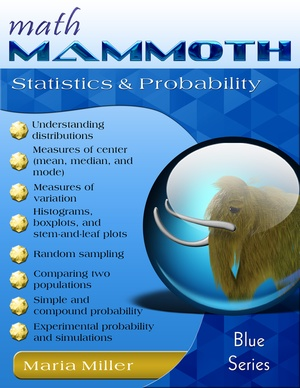You are here: HomeOnline resources → Probability

# Online games and resources for probability

This is an annotated and hand-picked list of online tutorials, games, worksheets, and activities for probability. I have tried to gather only the best, to make sure they are truly useful for my site visitors!

Probability   Books

## Probability

Probability lessons at Maths is Fun
Online lessons and exercises for simple probability, tree diagrams, independent & dependent events, combinations and permutations.
www.mathsisfun.com/data/index.html#stats

Math Goodies Probability Lessons
Tutorials with lots of worked-out examples and online interactive exercises. Topics include independent and dependent events, addition rules, conditional probability, and more.
www.mathgoodies.com/lessons/toc_vol6.html

Data Analysis & Probability Games from MathWire
A list of board and dice games for beginner probability topics.
mathwire.com/games/datagames.html

Free probability simulations for 7th grade
A set of four interactive probability simulations that use random digits in a spreadsheet file: die roller, two-coin toss, females/males in a sample of 10 people, and students who completed homework in a sample of 6 students.
mathmammoth.com/lessons/probability_simulations.php

Probability Game
A multiple-choice online quiz on simple probability
www.math-play.com/Probability-Game.html

A game played on a 100-chart of vines and toads. In level 1, answer questions about simple probability and get to move your piece. In level two, you work with the probability of two independent events.
www.learnalberta.ca

That Quiz: Probability
Online quizzes for the concept of simple (classic) probability.
www.thatquiz.org/tq-d/math/probability

Higher or Lower?
Bet on whether the next card shown will be higher or lower than the last one.
www.subtangent.com/maths/higher-lower.php

Coin Flip
A virtual coin toss. It shows the results numerically, and works for at least 100,000 flips.
www.btwaters.com/probab/flip/coinmainD.html

Dice Roll
A virtual dice roll. Choose the number of dice and how many rolls you want to do. The page shows both the actual and expected (theoretical) probabilities, and works for very large number of rolls.
www.btwaters.com/probab/dice/dicemain3D.html

Coin Toss Simulation
Another virtual coin toss. This one shows the results both using images of coins and numerically.
syzygy.virtualave.net/multicointoss.htm

Probability Practice
How likely is each ball to be picked? Impossible, unlikely, even chance, likely or certain?
www.bbc.co.uk/schools/teachers/ks2_activities/maths/probability.shtml

Virtual Experimental Probability
Roll die or two dice, spin a spinner, choose a card, or toss a coin virtually many times. The results are recorded so you can compare to theoretical probability.
staff.argyll.epsb.ca/jreed/math9/strand4/probability_display.htm

Probability Fair
Choose the probability that has the best chance when the colorful spinner is spun.
mrnussbaum.com/probfair-play

Cross the Bridge (PDF)
This is a printable board game based on throwing two dice and the probabilities for the sum of the dice.

Counting and Probability - from Interactive Mathematics
A full chapter of probability topics online, covering for example counting, basic probability, conditional probability, independent and dependent events, probability distributions, and more.
www.intmath.com/Counting-probability/Counting-probability-intro.php

Combinations and Permutations
A simple lesson from MathsIsFun.com.
www.mathsisfun.com/combinatorics/combinations-permutations.html

The Monty Hall Problem
Try this interactive version of the famous Monty Hall problem. Includes a simple explanation of the solution, as well. In the Monty Hall Problem you choose one of three closed doors. Behind one is a big prize and behind others something else, like a goat. After you choose a door, Monty opens one of the doors where there is no prize, and you have a chance to switch.
www.nytimes.com/2008/04/08/science/08monty.html?_r=1

Probability Interactive Activities
(click on 'probability' from the top menu)
Interactive tools for studying experimental and theoretical probability: an adjustable spinner, two regular 6-sided dice or customized dice.
www.shodor.org/interactivate/tools/

Probability Lessons
A set of free online lessons on various probability topics.
www.studygeek.org/statistics/

Visualizing Probabilites
This is an interesting article about modern visualisation techniques in the context of probabilities. The right picture really can be worth a thousand words.
plus.maths.org/content/understanding-uncertainty-visualising-probabilities

Probability Worksheets
Free printable worksheets on simple probability and statistics topics (middle school).
www.teach-nology.com/worksheets/math/stats

BooksMath Mammoth Statistics & Probability A worktext with both instruction and exercises, meant for grades 5-7. The book includes lessons on reading and drawing different graphs including circle graphs and stem-and-leaf plots, mean, median, mode, and range, and simple probability.Probability DemystifiedThis book is written as a self-teaching guide to adult audiences, and can be used as a high school book. It presents the basics of probability without formal proofs. The concepts will be developed from examples using coins, dice, cards, and other common probability devices. The book has problems at the end of each chapter and section, a 60-question "final exam", and answers to all problems.

You can find a chapter on probability in the following books as well:Mathematics, a Human Endeavor: A Textbook for Those Who Think They Don't Like the SubjectThough technically a textbook, this is excellent reading for anyone who's interested in learning about math. The subjects provide the reader with a broad introduction to the mathematical sciences, including geometry, probability, combinatorics, statistics, topology and more. More importantly, Jacob's examples and explanations make it relevant and fun.Challenge Math For the Elementary and Middle School StudentThere is a great lesson at the beginning followed by practice and then three levels of questions. The author has taken concepts that are generally saved for older kids (and can be dry and tedious) and made them accessible to a younger age group. Some of the concepts are fairly simple but as you work through how to apply them with increasing difficulty to some real-world problems then it does get you thinking.Precalculus with Trigonometry: Concepts and ConnectionsBy Paul Foerster. Following the line of his excellent textbooks for high school math.